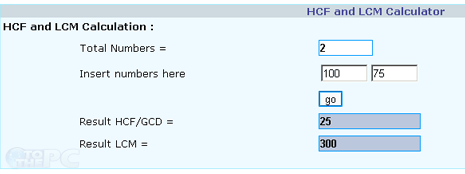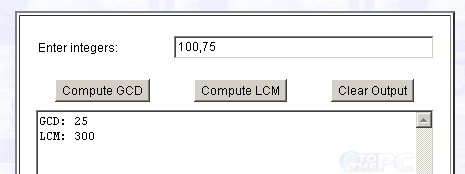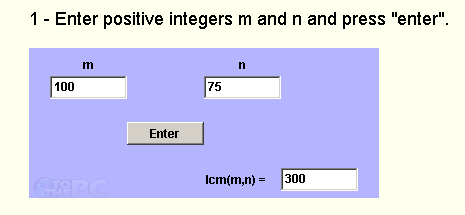# Calculate LCM, HCF, GCD of numbers online

For starters, LCM stands for Least Common Multiple while HCF stands for Highest Common Factor and GCD stands for Greatest Common Divisor. These are important terms used in mathematical calculation of numbers. Are you looking for LCM / HCF online calculators for quick and easy calculation of LCM, HCF and GCD values of given number? Following resources should be of great help.

## 1. Calculator at Easy Calculation websiteHead over to Easy Calculation website to quickly calculate LCM and HCF of given numbers. To get started, enter total of input numbers and then enter actual numbers for calculation. Click GO button to get HCF/GCD and LCM result for specified numbers.

## 2. GCD / LCM online calculatorGCD/LCM calculator is an online tool for quick calculation of GCD and LCM values from specific numbers. Enter integer values separated by commas, then click ‘compute GCD’ and ‘compute LCM’ to get respective GCD and LCM results.

## 3. AnalyzeMath’s LCM, GCF toolsIf you want to calculate LCM and GCF values between only two integer numbers, then check online calculator tools at Analyze Math website. Input two numbers and click Enter button to get LCM value and GCF value for those numbers.

SHARE